# 2nd PUC Electronics Model Question Paper 2 with Answers

Students can Download 2nd PUC Electronics Model Question Paper 2 with Answers, Karnataka 2nd PUC Electronics Model Question Papers with Answers helps you to revise the complete Karnataka State Board Syllabus and score more marks in your examinations.

## Karnataka 2nd PUC Electronics Model Question Paper 2 with Answers

Max Marks: 70
Time : 3 hrs. 15min.

Part – A

Answer all questions: 10 x 1 = 10

Question 1.
Define pinch-off voltage.
Pinch off voltage is the gate voltage at which drain current becomes almost constant.

Question 2.
Define quiescent point.
Q point is the zero signal values of collector current Ic and collector to emitter voltage VCE.

Question 3.
Name any one material which exhibits piezo electric effect.
QuartzQuestion 4.

Question 5.
How many side bands are present in AM wave?
Two side bands.

Question 6.
What is frequency modulation?
Frequency modulation is varying the frequency of the carrier wave in accordance with the modulating signal.

Question 7.
Define a QUAD in a K-map.

Question 8.
Convert 1111 to gray code.
(1111 )2 = (1000) in Gray codeQuestion 9.
What does a ‘jump’ instruction do in microcontroller programming?
Jump permanently changes the contents of the program counter if centain program condition exists.

Question 10.
What is the value of 17% – 2 in C programming?
1

Part -B

Answer any five questions. 5 x 2 = 10

Question 11.
What is a heat sink? Mention its use.
Heat sink is a device which absorbs the unnecessary heat generated in the transistor and radiates it to surroundings and protects it.

Question 12.
Draw the frequency response of a CE amplifier.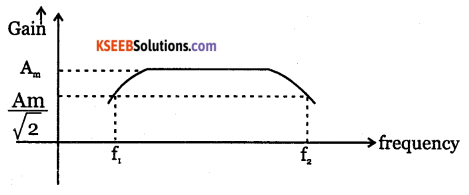Question 13.
Write any four characteristic features of voltage series negative feedback amplifier.

• Voltage gain decreases
• Input impedance increases
• Output impedance decreases
• Bandwidth increases.Question 14.
Name the four different modes of a differential amplifier.
Differential amplifier is a circuit which amplifies the difference of inputs applied to it.Dual input and balanced output differential Amplifier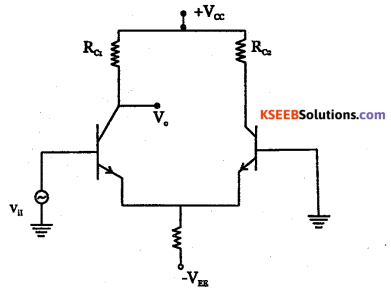Single input and unbalanced output differncial amplifier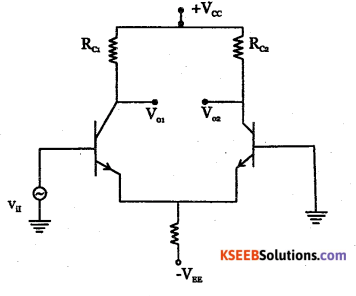Single input and balanced output differncial amplifierDual input and unbalanced output differential AmplifierQuestion 15.
Distinguish between damped and undamped oscillations.

• Damped oscillations are electrical oscillations whose amplitude keeps on decreasing with time but frequency remains a constant.
• Undamped oscillations are electrical oscillations whose amplitude remains constant with respect to time.

Question 16.
Mention different opcodes used in 8051.
MOV, MOVX, MO VC, PUSH and POP, XCH

Question 17.
What is the use of main ( ) function in C?
Main ( ) is a special function used by C system to tell the computer where the program starts.

Question 18.
Expand AMPS and TDMA.
AMPS = Advanced mobile phone service
TDMA=Time Division Multiple Access.

Part – C

Answer any five questions. 5 x 3 = 15

Question 19.
Give a comparison between FET and BJT.

 FET BJT It is a voltage controlled device. It is a current controlled device. It is a unipolar device. It is a bipolar device.Question 20.
Draw the frequency response of an amplifier with and without feedback and comment
on the gainbandwidth product of the amplifier.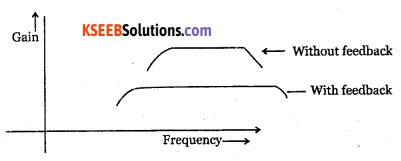Question 21.
Explain in brief the ground wave propagation.
Ground waves are the waves transmitted along or reflected from the surface of the earth. These can exist when the transmitting and receiving antennas are close to the surface of the earth.

The ground wave is vertically polarised and will transmit most effectively at a frequency less than 2 MHZ. The AM broad cast signals travel as ground waves. The strength of the ground wave is reduced due to energy absorption by the earth’s surface.

Question 22.
Show that the total power in an AM wave is 3/2 times the carrier power.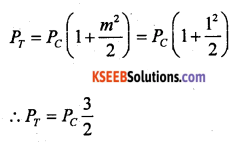Question 23.
Explain the terms sensitivity, selectivity and fidelity with respect to a radio receiver.

• Sensitivity of a radio receiver is its ability to respond to weak signals.
• Selectivity is the ability of a radio receiver to distinguish between desired signal to which the receiver is tuned and the other signal frequencies.
• Fidelity of a radio receiver is its ability to reproduce faithfully in its output, the signal that appears at its input.

Question 24.
Explain briefly the working of non-punch through diode with its electric field profile
diagram.
In non-punch through diodes, the depletion region boundary does not reach the end of drift layer. In punch through diode, the depletion layer spans the entire drift region and is in contact with n+ cathode.Question 25.
Write the classification of RADAR systems.

• Moving target indicator (MTI) radar

Question 26.
Draw the logic diagram of PISO register. Explain the SHIFT / $$\overline{L O A D}$$ action in it.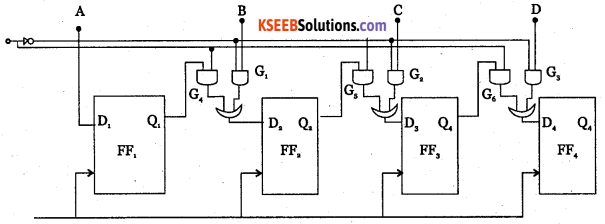Part – D

Answer any five questions. 3 x 5 = 15

Question 27.
Calculate the voltage gain, input impedance and output impedance of a CE amplifier with IE = 1.3 in mA, β = 100, Rc = 10kQ, RL = 10kΩQuestion 28.
Calculate the output voltage VQ.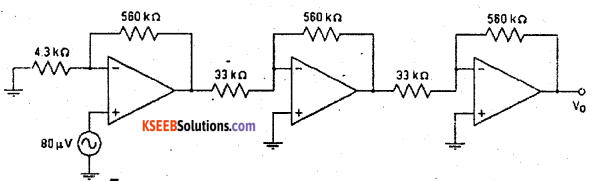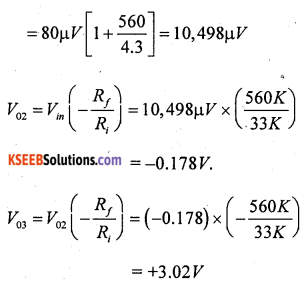Question 29.
A Hartley oscillator circuit is to generate a frequency of 1200 kHz. If the capacitor in the feedback network has a value of 220 pF and one of the inductors value is 20 pH, calculate the value of the other inductor.Question 30.
Simplify using K-map Y(A, B, C, D) = Em (2, 4, 5, 9,10, 12,14, 15) + Ed (0, 6, 8,13). Realize the simplified expression using NAND gates only.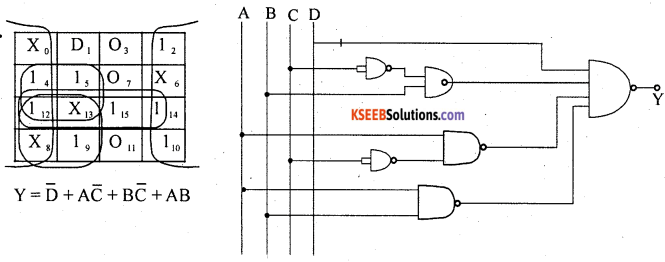Question 31.
Write a program to multiply two 8 bit numbers 06H and 09H at memory locations 40H and 41H respectively. Store the result at memory locations 42H (Lower Byte) and 43H (Higher Byte).
Mov a,  40
Mov fo,  41
Mul a,  b
Mov 42,  a
Mov 43,  fo

Part – E

Answer any Four questions. 4 x 5 = 20

Question 32.
Give a comparison of different power amplifiers.

 Class A B C AB Conduction angle 360° 180° Less than 90° 180° to 360° Position of Q point Centre of load line. on x-axis (cut off) Below x-axis Between x-axis and centre of load line Overall efficiency 25% 70-80% Higher than 80% 50-70% Signal distortion None At x-axis cross over point Large Amounts Small amounts.Question 33.
With a relevant diagram, derive an expression for the output voltage of an op-amp logarithmic amplifier.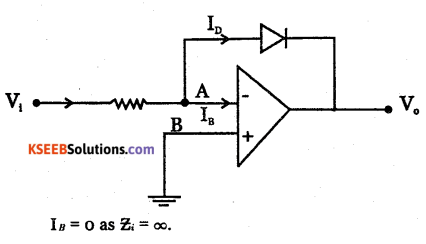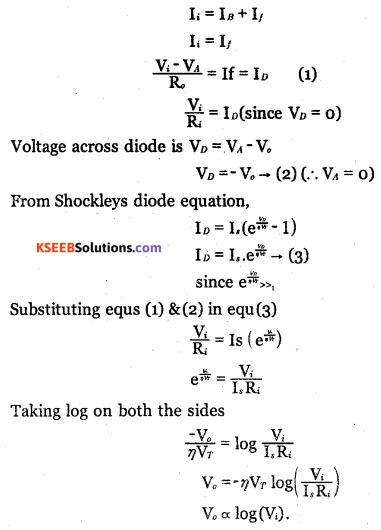∴ The output voltage is proportional to natural logarithm of input voltage.Question 34.
Write the block diagram of digital communication and explain the function of each block.
Source encoder: Converts analog signal into binary code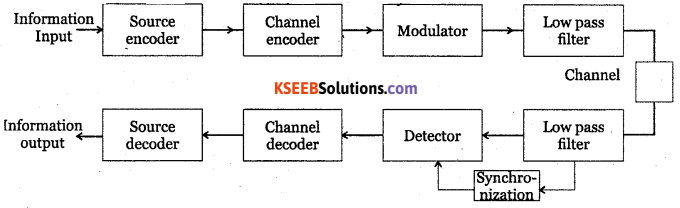• Channel encoder: Generates channel code word from the source code words.
• Modulator: Modulates the channel code words into a continuous wave.
• Low pass filter: Allows only lower frequencies and blocks the other frequencies.
• Synchronisation: Is required to demodulate a carrier modulated wave.
• Demodulator: Converts continuous waveform into ordinary signal.
• Channel decoder: Decodes the channel code word.
• Source decoder: Converts channel decoder code word into information signal.

Question 35.
What is a full adder? Explain its working with respect to three input X – OR gate and basic gates with the help of truth table and Boolean expression.
Full adder is a combinational logic circuit that performs arithmetic sum of three bits and gives their sum and carry .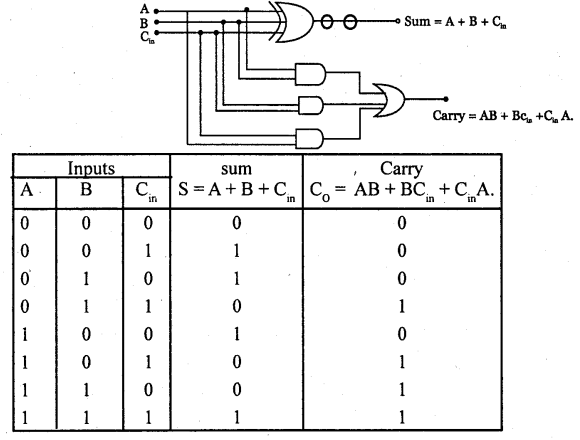Question 36.
With circuit diagram, explain the working of single phase SCR half wave rectifier with RC triggering.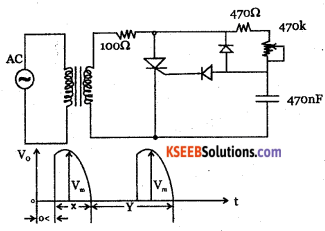The load is connected before RC circuit. In this circuit the capacitor voltage reduces, when SCR is conducting. Diode D2 along with D1 is used to prevent negative voltage to the gate w. r. t. the cathode. The firing angle for half wave rectifier is
$$\alpha=180-\left(\frac{x}{\frac{y}{2}}\right) \times 180$$Question 37.
What is a variable in C language? Mention the rules for constructing variable names in C.